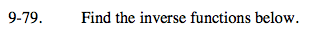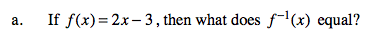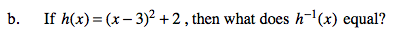Home > A2C > Chapter 9 > Lesson 9.2.1 > Problem9-79

9-79.
1. Find the inverse functions below. Homework Help ✎

1. If f(x) = 2x − 3, then what does f 1(x) equal?

2. If h(x) = (x − 3)2 + 2, then what does h1(x) equal?x = 2f −1(x) − 3
Solve for f −1(x).

$f^{-1}(x)=\frac{{x+3}}{2}$See part (a).

$h^{-1}(x)=\sqrt{x-2}+3$